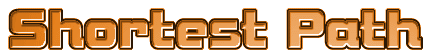(single source)Uneighted GraphBreath - First SearchWeighted Graph : Dijkstra's algorithm

S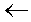{v0};    for each vV do D[v]d(v0,v);                       *
while  S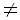V do{
choose w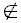S , D[w] min;
SS U {w};
for each vV-S  do                        *
D[v]min(D[v] , D[w]+d[w,v]);
}

Property :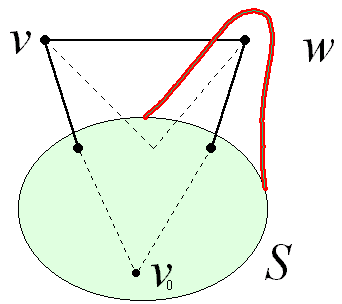v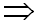D[v] = shortest path from v0 to v
vD[v] = shortest path from v0 to v through x (in S)
proof : othewise ,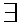a path which is the combination of the path
from v0 to v and from v to wExample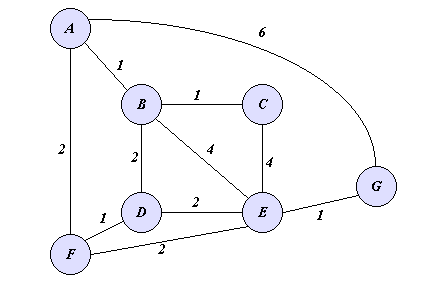S A B C D E F G A 0 1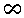2 6 A   B 0 1 2 3 5 2 6 A   B   F 0 1 2 3 4 2 6 A   B   C    F 0 1 2 3 4 2 6 A   B   C    D   F 0 1 2 3 4 2 6 A   B   C    D   E   F 0 1 2 3 4 2 5 A   B   C    D   E   F   G 0 1 2 3 4 2 5For both directed and undirected graphT = O(N2)Priority-First-Search implementation : Priority = D[w]+d(w , v)Priority queue =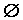; put(S);Only modify D[v] , for w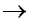v